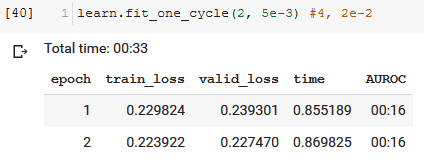# Using AUC as metric in fastai

You can use it as a metric, but not as a loss function. Just search this forum or Kaggle kernels for code examples.

Because AUROC yields a number from a set of predictions, it is not differentiable, and therefore cannot be directly optimized as a loss function. “Dice loss” is said to be a way to approximate AUROC. I have not yet experimented with it. You can find many PyTorch code examples via Google.

2 Likes

Unfortunately, I could not find any code examples for the ROC curve in fastai.
I am using the Dice coefficient as a metric, but I am not sure that is what you mean.

Here you can see my notebook. Should I aim for a dice of zero or one?

Have a look at the forum search results for `roc_auc_score`

1 Like

This kernel (https://www.kaggle.com/quanghm/fastai-1-0-tabular-learner) implements one based on Scikit-learn’s auc score and it seems to work. More examples on creating your own: https://docs.fast.ai/metrics.html
Update: I came across cases where the auc metric will fail if (I believe) the batch has one class as output. In this case, increasing the batch size (default 64) may work.

3 Likes

Olá João,

Dice can be used as a loss function instead of the default cross-entropy loss. It may then optimize for a higher AUROC than cross-entropy loss does. At least some posters think so. You can find lots of opinions and methods by searching for “PyTorch dice loss” in Google. For myself and for now, the default cross-entropy loss serves well enough for optimizing AUROC.

It might help you to study the difference between metric and loss function. Not very long ago I was also unclear about this distinction! They are discussed in both the Lessons and the forum.

If you try both cross-entropy loss and dice loss on your problem, I would be interested to know how the resulting AUROCs compare.

3 Likes

I faced this requirement a couple of weeks ago.

Fastai computes metrics for each batch and then averaged across all batches, which makes sense for most metrics. However, AUROC can not be computed for individual batches, requiring to be computed on the entire dataset at once.

So, I implemented a callback to compute the AUROC:

``````from sklearn.metrics import roc_auc_score

def auroc_score(input, target):
input, target = input.cpu().numpy()[:,1], target.cpu().numpy()
return roc_auc_score(target, input)

class AUROC(Callback):
_order = -20 #Needs to run before the recorder

def __init__(self, learn, **kwargs): self.learn = learn
def on_epoch_begin(self, **kwargs): self.output, self.target = [], []

def on_batch_end(self, last_target, last_output, train, **kwargs):
if not train:
self.output.append(last_output)
self.target.append(last_target)

def on_epoch_end(self, last_target, last_output, **kwargs):
if len(self.output) > 0:
output = torch.cat(self.output)
target = torch.cat(self.target)
preds = F.softmax(output, dim=1)
metric = auroc_score(preds, target)
``````

Then you should pass the callback to the learner. In my case:

`learn = text_classifier_learner(data_clf, drop_mult=0.3, callback_fns=AUROC)`

Finally, when you train, you get something like this:

Hope it helps.

Side note: it was the first time for me implementing a callback and I got surprised at how easy fastai makes these kinds of customizations.

35 Likes

@sgugger: Would it be worth to add this feature to fastai?

5 Likes

Double check that it works properly against an existing implementation (like in scikit-learn) then yes, definitely suggest a PR! Thanks!

3 Likes

Thanks for this! I was wondering the same thing - whether it’s valid to average AUROC over minibatches - and did not know how to implement the right way.

FYI, I implemented this metric the wrong way - where it’s averaged over minibatches - and have been using it. However, by this accidental experiment, I saw it gives about the same answer whether averaged over minibatches or done over the validation set all at once. Within .1%, and apparently less over more minibatches. There’s likely a theorem lurking around here.

3 Likes

Also, I embarrassingly posted an incorrect version of the AUROC metric a couple of months ago in a different thread. That function was missing softmax, which you need to first apply across the two class activations sent to the fastai metric. In the context of Validation set predictions however (learn.get_preds), softmax is automatically applied, so the positive class probability gets passed directly to roc_auc_score.

Thank you very much to all of you, this thread was very helpful. I ended up using joshpf’s implementation.

1 Like

I think there is a small issue with the above metric used as callback (not sure if applies to all or if being fixed - using current master). The added `time` column header should be the last one.Oh, that’s a bug. Let me fix it!

Edit: Done in master

2 Likes

Is this callback function applicable to any leaner? - for e.g. a tabular learner?
I’ve tried to just use this piece of code - and nothing shows up under AUROC

What should I change? I’m a noob - pardon my ignorance

Is it a binary classifier?

I think I just made the exact same mistake- the code snippet José provided is slightly cut off and you have to scroll, there’s one additional line at the end of the on_epoch_end() function:

``````    def on_epoch_end(self, last_target, last_output, **kwargs):
if len(self.output) > 0:
output = torch.cat(self.output)
target = torch.cat(self.target)
preds = F.softmax(output, dim=1)
metric = auroc_score(preds, target)
``````

Hey @joshfp,

I’m trying to use your class for tabular_learner (binary classification), but I’m getting the following error:
`AttributeError: 'Learner' object has no attribute 'add_metrics'`
Do you have any idea why I’m getting this error?

Thanks!
David

I faced the same issue. Worked for me with the following change

``````def on_epoch_end(self, last_metrics, **kwargs):
if len(self.output) > 0:
output = torch.cat(self.output)
target = torch.cat(self.target)
preds = F.softmax(output, dim=1)
metric = auroc_score(preds, target)